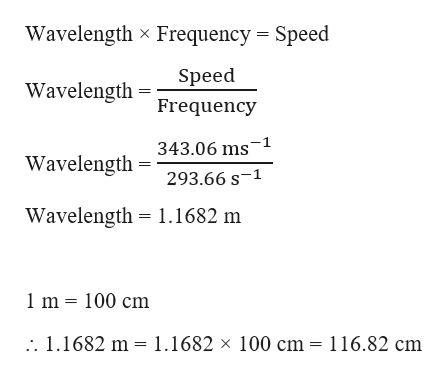# The frequency of the middle D note on a piano is 293.66 Hz. What is the wavelength of this note in centimeters? The speed of sound in air is 343.06 m/s.

Question

The frequency of the middle D note on a piano is 293.66 Hz. What is the wavelength of this note in centimeters? The speed of sound in air is 343.06 m/s.

check_circle

Step 1

Given,

Frequency of sound = 293.66 Hz = 293.66 s-1

Speed of sound = 343.06 m s-1

The wave...help_outlineImage TranscriptioncloseWavelength x Frequency Speed Speed Wavelength Frequency 343.06 ms 1 Wavelength 293.66 s-1 Wavelength 1.1682 m 1m 100 cm 1.1682 m 1.1682 x 100 cm = 116.82 cm fullscreen

### Want to see the full answer?

See Solution

#### Want to see this answer and more?

Solutions are written by subject experts who are available 24/7. Questions are typically answered within 1 hour.*

See Solution
*Response times may vary by subject and question.
Tagged in

### Chemistry